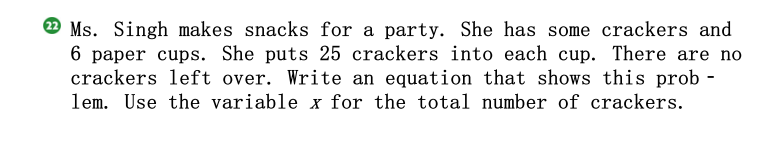### ¿Todavía tienes preguntas de matemáticas?

Pregunte a nuestros tutores expertos
Algebra
Pregunta(22) Ms. Singh makes snacks for a party. She has some crackers and

$$6$$ paper cups. She puts $$25$$ crackers into each cup. There are no crackers left over. Write an equation that shows this prob- lem. Use the variable $$x$$ for the total number of crackers.

$$x= 6*25$$